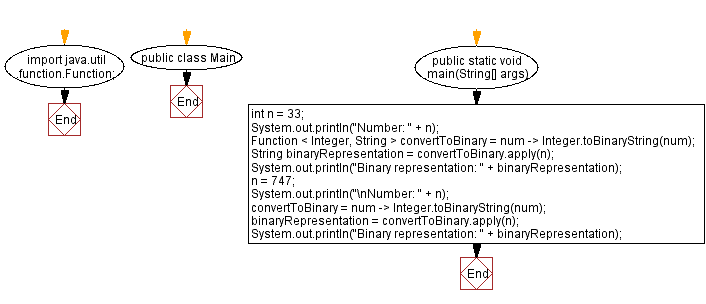# Java Program: Lambda expression to convert integer to binary

## Java Lambda Program: Exercise-25 with Solution

Write a Java program to implement a lambda expression to convert an integer to their corresponding binary representation.

Sample Solution:

Java Code:

``````import java.util.function.Function;

public class Main {
public static void main(String[] args) {
int n = 33;
System.out.println("Number: " + n);

Function < Integer, String > convertToBinary = num -> Integer.toBinaryString(num);
String binaryRepresentation = convertToBinary.apply(n);
System.out.println("Binary representation: " + binaryRepresentation);
n = 747;
System.out.println("\nNumber: " + n);
convertToBinary = num -> Integer.toBinaryString(num);
binaryRepresentation = convertToBinary.apply(n);
System.out.println("Binary representation: " + binaryRepresentation);
}
}
``````

Sample Output:

```Number: 33
Binary representation: 100001

Number: 747
Binary representation: 1011101011
```

Explanation:

In the above exercise the program uses the Integer.toBinaryString() method to convert an integer to its binary representation. The lambda expression allows you to encapsulate this conversion logic and use it as a function.

The lambda expression:

• It takes an integer as input (num).
• It uses the Integer.toBinaryString() method to convert the integer to its binary representation.
• It returns the binary representation as a string.

Flowchart:Live Demo:

Java Code Editor:

Improve this sample solution and post your code through Disqus

Java Lambda Exercises Previous: Lambda expression to find largest prime factor.

What is the difficulty level of this exercise?

Test your Programming skills with w3resource's quiz.

﻿

## Java: Tips of the Day

How do I remove repeated elements from ArrayList?

If you don't want duplicates in a Collection, you should consider why you're using a Collection that allows duplicates. The easiest way to remove repeated elements is to add the contents to a Set (which will not allow duplicates) and then add the Set back to the ArrayList:

```Set<String> set = new HashSet<>(yourList);
yourList.clear();